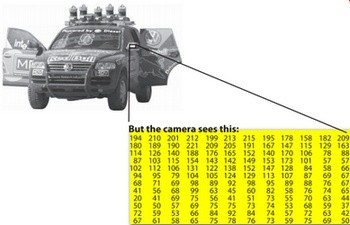前言

Mat 是Opencv中很常用的一个图像容器类，图像在计算机中的存储形式是二进制字节流，其本质的存储形式如下图所示；而一张图片是由很多像素点组成，单个像素点又会因为图像格式的不同而不同。例如彩色的RBG或者灰度图像。而在OpenCV中，则可以抽象成一个顺序排列的内存区域，里面保存了图像的所有像素信息，这里用Mat类封装了这些图像的信息，包括图像大小，类型等等，大大地简化了我们处理和操作图像。

概念

Mat 是一个类，从最早的OpenCV是C语言风格发展到现在的C++风格，它对面向对象的支持更加友好。相比较于之前C结构的IplImage，Mat有更多有优点；

1. 内存的分配与释放更加安全；
2. 使用Mat可以使代码更加简洁；
3. 对于图像数据的处理更加高效；

Mat基本上是一个包含两个数据部分的类：矩阵头（包含矩阵大小，用于存储的方法，存储矩阵的地址等信息）和指向包含矩阵的矩阵的指针。像素值（取决于选择存储的方法取任何维度）。矩阵头大小是恒定的，但是矩阵本身的大小可能随图像而变化，并且通常大几个数量级。OpenCV是一个图像处理库。它包含大量图像处理功能。为了解决计算挑战，大多数时候您最终会使用库的多个功能。因此，将图像传递给函数是一种常见的做法。我们不应该忘记我们正在讨论图像处理算法，这些算法往往计算量很大。我们要做的最后一件事是通过制作不必要的潜在大图像副本来进一步降低程序的速度。为解决此问题，OpenCV使用引用计数系统（Reference Counting System）。这个想法是每个Mat对象都有自己的头，但是矩阵可以通过让它们的矩阵指针指向同一个地址来共享它们的两个实例。此外，复制操作符只会将标题和指针复制到大矩阵，而不是数据本身。

实战

1 基础操作

1初始化

//CV_8UC(n), ..., CV_64FC(n)
Mat A(5,5,CV_64FC1,1);
std::cout << "A= " << endl << " " << A << endl;

Mat B(5,5,CV_64FC1,2);
std::cout << "B= " << endl << " " << B << endl;

Mat C(5,5,CV_64FC3,Scalar(6.0f,7.0f,8.0f));
std::cout << "C= " << endl << " " << C << endl;

Mat D = Mat::eye(3,3,CV_64F);
std::cout << "D= " << endl << " " << D << endl;

Mat E = Mat::ones(3,3,CV_64F);
std::cout << "E= " << endl << " " << E << endl;

Mat F = Mat::zeros(3,3,CV_64F);
std::cout << "F= " << endl << " " << F << endl;
```c
>A=
[1, 1, 1, 1, 1;
1, 1, 1, 1, 1;
1, 1, 1, 1, 1;
1, 1, 1, 1, 1;
1, 1, 1, 1, 1]
B=
[2, 2, 2, 2, 2;
2, 2, 2, 2, 2;
2, 2, 2, 2, 2;
2, 2, 2, 2, 2;
2, 2, 2, 2, 2]
C=
[6, 7, 8, 6, 7, 8, 6, 7, 8, 6, 7, 8, 6, 7, 8;
6, 7, 8, 6, 7, 8, 6, 7, 8, 6, 7, 8, 6, 7, 8;
6, 7, 8, 6, 7, 8, 6, 7, 8, 6, 7, 8, 6, 7, 8;
6, 7, 8, 6, 7, 8, 6, 7, 8, 6, 7, 8, 6, 7, 8;
6, 7, 8, 6, 7, 8, 6, 7, 8, 6, 7, 8, 6, 7, 8]
D=
[1, 0, 0;
0, 1, 0;
0, 0, 1]
E=
[1, 1, 1;
1, 1, 1;
1, 1, 1]
F=
[0, 0, 0;
0, 0, 0;
0, 0, 0]
## 2 读取图片

```c
#include <iostream>
#include <opencv2/opencv.hpp>

int main(char argc,char** argv){
cv::Mat img = cv::imread(argv,-1);
if(img.empty()){
return -1;
}
cv::imshow("Image",img);
cv::waitKey( 0 );
return 0;
}

3 图像ROI

#include <iostream>
#include <opencv2/opencv.hpp>
#include <opencv2/highgui.hpp>

using namespace cv;
using namespace std;

int main(int argc,char* argv[]){